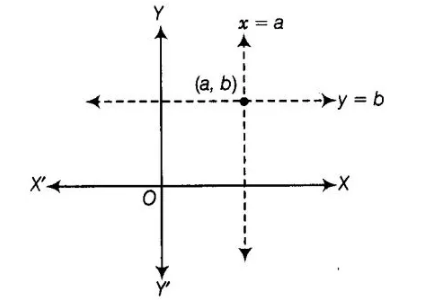# The pair of equations x = a and

Question:

The pair of equations x = a and y = b graphically represents lines which are

(a) parallel

(b) intersecting at (b, a)

(c) coincident

(d) intersecting at (a, b)

Solution:

(d) By graphically in every condition, if a, b>>0; a, b< 0, a>0, b< 0; a<0, b>0 but a = b≠ 0.

The pair of equations x = a and y = b graphically represents lines which are intersecting at (a, b).

If a, b > 0Similarly, in all cases two lines intersect at (a, b).